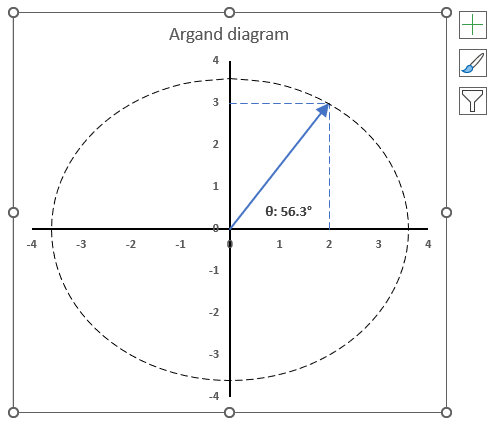Author: Oscar Cronquist Article last updated on May 19, 2023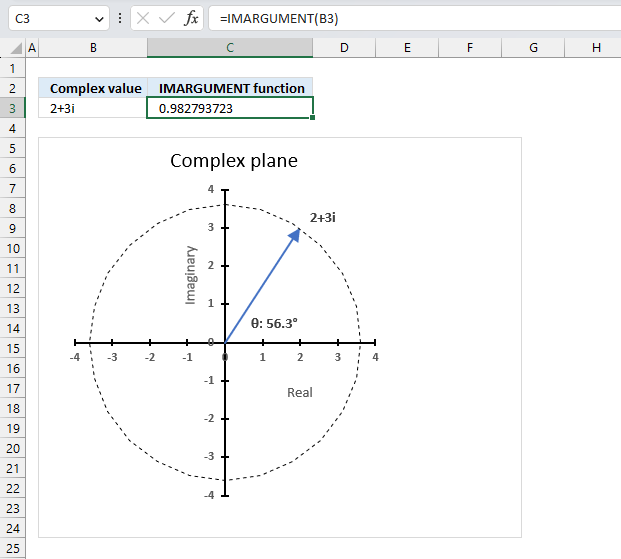The IMARGUMENT function calculates the argument theta θ which is an angle displayed in radians based on complex numbers in rectangular form like z = x + yi or z = x + yj.

## 1. IMARGUMENT Function Syntax

IMARGUMENT(inumber)

## 2. IMARGUMENT Function Arguments

 inumber Required. A complex number in x+yi or x+yj text format.

## 3. IMARGUMENT function exampleThe image above shows how to calculate the θ with the IMARGUMENT function based on the corresponding complex numbers on the same row specified in cell B3. The dashed circle represents the complex modulus |z| of "2+3i" and the angle theta represents its complex argument.

Formula in cell C3:

=IMARGUMENT(B3)

The IMARGUMENT function returns a value expressed in radians, the image above shows a chart where theta θ is displayed in degrees.

### 3.1 Explaining formula

#### Step 1 - Populate arguments

IMARGUMENT(inumber)

becomes

IMARGUMENT(B3)

#### Step 2 - Evaluate IMARGUMENT function

IMARGUMENT(B3)

becomes

IMARGUMENT("2+3i")

and returns

0.982793723247329

## 4. How is the theta θ calculated in detail?The IMARGUMENT function calculates theta θ expressed in radians using this formula:

θ = tan-1(y/x) for x>0

Here is how 0.982793723247329 is calculated:

tan θ = y/x

becomes

θ = tan-1(y/x)

tan-1(y/x)

becomes

tan-1(3/2)

equals

0.982793723247329

## 5. How to convert angle theta θ from radians to degreesThe following formula calculates theta θ based on a given complex number in rectangular form, the result is a value expressed in radians.

The DEGREE function takes the radian value and converts it to degrees.

Formula:

=DEGREES(IMARGUMENT(B3))

### 5.1 Explaining formula

#### Step 1 - Calculate theta θ in radians

The IMARGUMENT function calculates theta θ which is an angle displayed in radians based on complex numbers in rectangular form.

Function syntax: IMARGUMENT(inumber)

IMARGUMENT(B3)

becomes

IMARGUMENT("2+3i")

and returns

0.982793723247329

#### Step 2 - Convert radians to degrees

The DEGREES function calculates degrees from radians.

Function syntax: DEGREES(angle)

DEGREES(IMARGUMENT(B3))

becomes

DEGREES(0.982793723247329)

and returns

56.3099324740202

## 6. How to plot theta θ - Argand diagram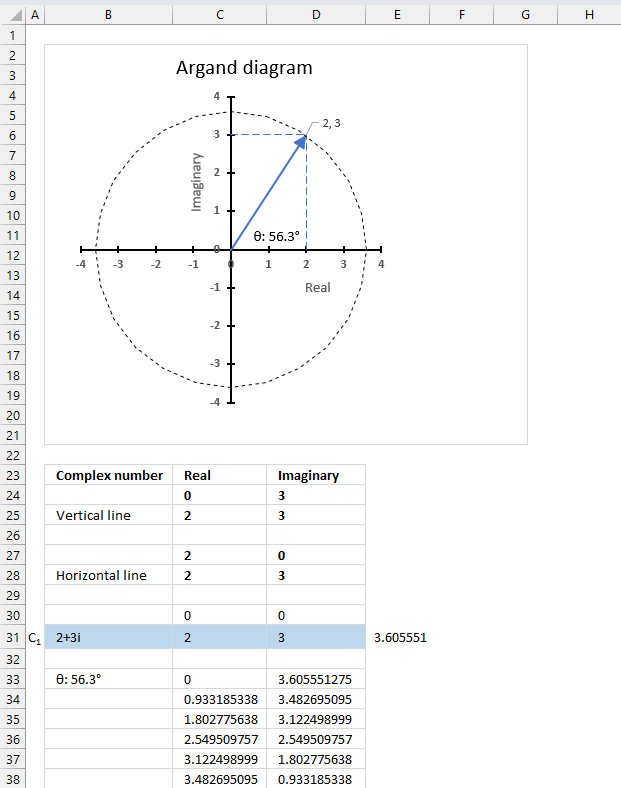The image above shows an Argand diagram which is a chart of complex numbers, the dashed circle is the modulus of the complex numbers.

C1 = 2  +3i

|C1| = |2  +3i| = Modulus = square root of 13 = 3.605551

theta θ = tan-1(3/2) = 0.982793723247329 radians = 56.3099324740202 degrees

Change the complex number in cell B31 and the chart adjusts accordingly.

### 6.1 Calculate the numbers

We need to setup the worksheet before we can insert the scatter chart.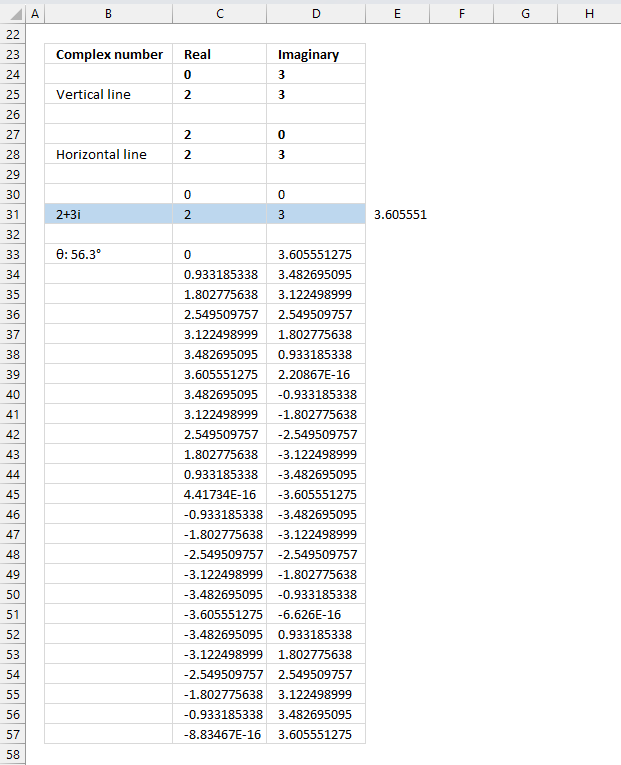Value in cells C24, D27, C30, and D30: 0

Formula in cell D24, D25, D28, and D31:

=IMAGINARY(B31)

Formula in cell C25. C27, C28, and C31:

=IMREAL(B31)

Value in cell D27: 0

Value in cells C30 and D30: 0

Formula in cell B33:

="θ: "&ROUND(DEGREES(ATAN(D31/C31)),1)&"°"

### 6.2 Explaining formula in cell B33

The value in cell B33 will be used in the chart, I will show you how to do that below.

#### Step 1 - Calculate theta θ

The ATAN function calculates the arctangent of a number.

Function syntax: ATAN(number)

ATAN(D31/C31)

#### Step 2 -  Convert radians to degree

The ATAN function calculates the arctangent of a number.

Function syntax: ATAN(number)

DEGREES(ATAN(D31/C31))

#### Step 3 - Round degrees to one digit

The ATAN function calculates the arctangent of a number.

Function syntax: ATAN(number)

ROUND(DEGREES(ATAN(D31/C31)),1)

#### Step 4 - Concatenate values

"θ: "&ROUND(DEGREES(ATAN(D31/C31)),1)&"°"

### 6.3 Create values for a circle on the chart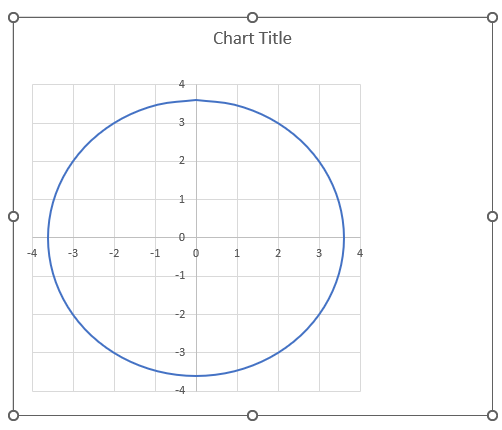The following formulas in cells C33 and D33 calculates values that creates a circle on the chart, the image above shows a circle on a chart.

Formula in cell C33:

=SIN(PI()/12*(ROWS(\$A\$1:A1)-1))*IMABS(\$B\$31)

### 6.4 Explaining formula in cell C33

#### Step 1 - Calculate each 15 degree segment

The PI function returns the number pi (¶).

Function syntax: PI()

PI()/12

#### Step 2 - Create a sequence

The ROWS function calculate the number of rows in a cell range.

Function syntax: ROWS(array)

ROWS(\$A\$1:A1)-1

#### Step 3 - Multiply segment with sequence

The ROWS function calculate the number of rows in a cell range.

Function syntax: ROWS(array)

PI()/12*(ROWS(\$A\$1:A1)-1)

#### Step 4 - Calculate sine

The SIN function calculates the sine of an angle.

Function syntax: SIN(number)

SIN(PI()/12*(ROWS(\$A\$1:A1)-1))

#### Step 5 - Calculate modulus

The IMABS function calculates the absolute value (modulus) of a complex number in x + yi or x + yj text format.

Function syntax: IMABS(inumber)

IMABS(\$B\$31)

#### Step 6 - Multiply sine with modulus

SIN(PI()/12*(ROWS(\$A\$1:A1)-1))*IMABS(\$B\$31)

Formula in cell D33:

=COS(PI()/12*(ROWS(\$A\$1:A1)-1))*IMABS(\$B\$31)

The formula in cell D33 is the same as in cell C33, except it calculates the cosine instead.

The cos function calculates the cosine of an angle.

Function syntax: COS(number)

Copy cells C33 and D33, paste to 24 rows below so the entire circle can be charted.

### 6.5 Insert "Scatter with smooth lines" chart

1. Select cell range B24:D57.
2. Go to tab "Insert" on the ribbon.
3. Press with left mouse button on the "Scatter (x,y) or Bubble chart" button on the ribbon.
4. A popup menu appears. Press with left mouse button on the "Scatter with smooth lines" button.

A new chart is now created. Move the chart to the desired location.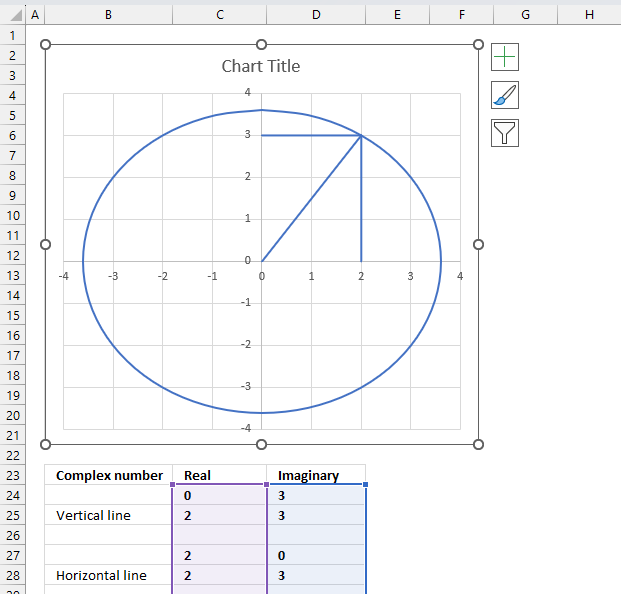Select the chart, the boundaries now have "handles" that you can drag with the mouse to resize the chart.

1. Double-press with left mouse button on the circle to select all lines on the chart and open the settings pane.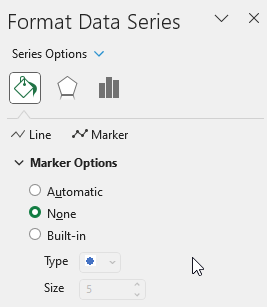2. Press with left mouse button on the "Fill & Line" button on the settings pane.
3. Press with left mouse button on the "Line" on the settings pane, new settings in context to "Line" shows up.
4. Press with left mouse button on the "Dash type" button, select a dashed line.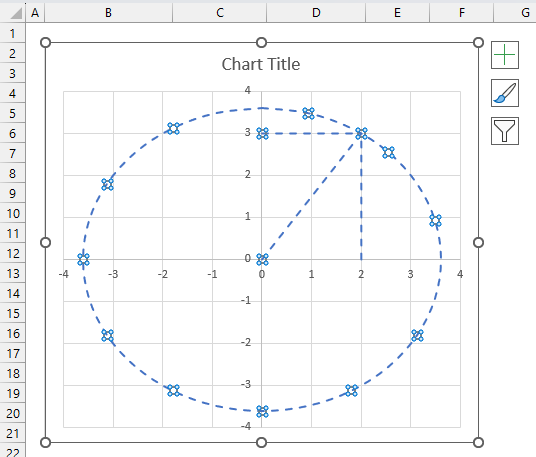5. All lines are now dashed. Press with left mouse button on the "Color" button and change to black.

### 6.6 Change the complex number line on the chart

1. Select only the "complex number" line. Press with left mouse button on the "complex number" line once to select it if you have all lines selected.
If no lines are selected then press with left mouse button on the "complex number" line twice to select it.
2. Press with left mouse button on the "Line" on the settings pane. Change color, dash type and add an ending arrow.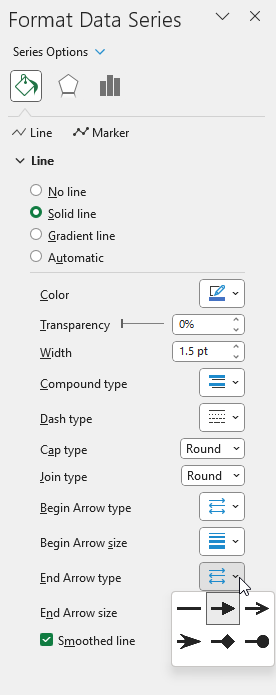3. Repeat these steps for the imaginary and real lines.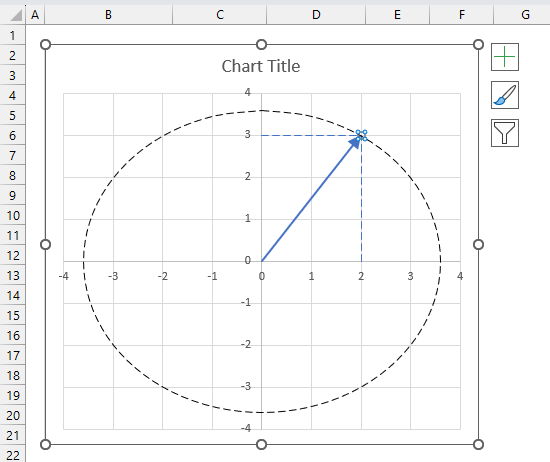Change the chart tite, use the settings pane to change the axis line widths and colors, remove chart grids.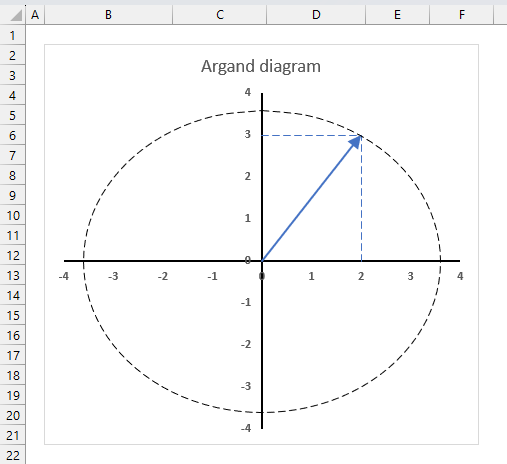1. Select the "complex number" line (blue).
2. Press with left mouse button on the "plus" sign next to the chart.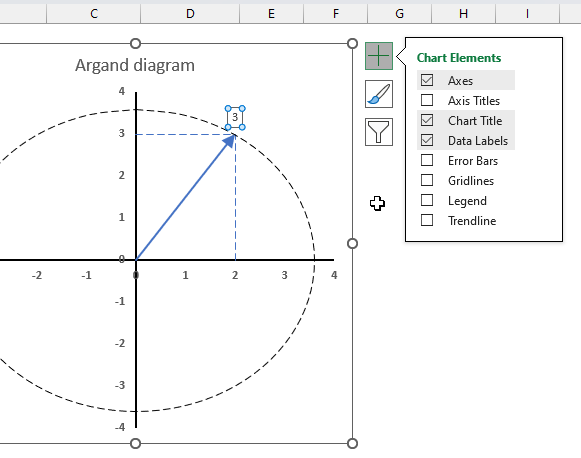3. Press with left mouse button on the check box next to "Data Labels" to enable data labels.
4. Open the settings pane.
5. Go to "Label options"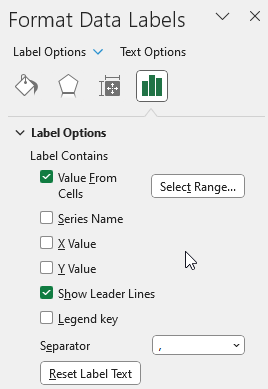6. Press with left mouse button on checkbox next to "Value From Cells", select cell B33.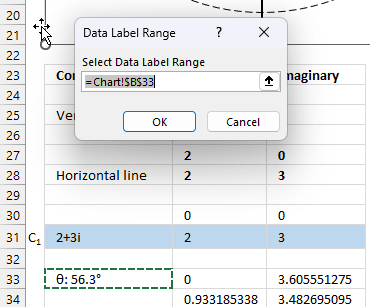7. Move the data label to its destination.